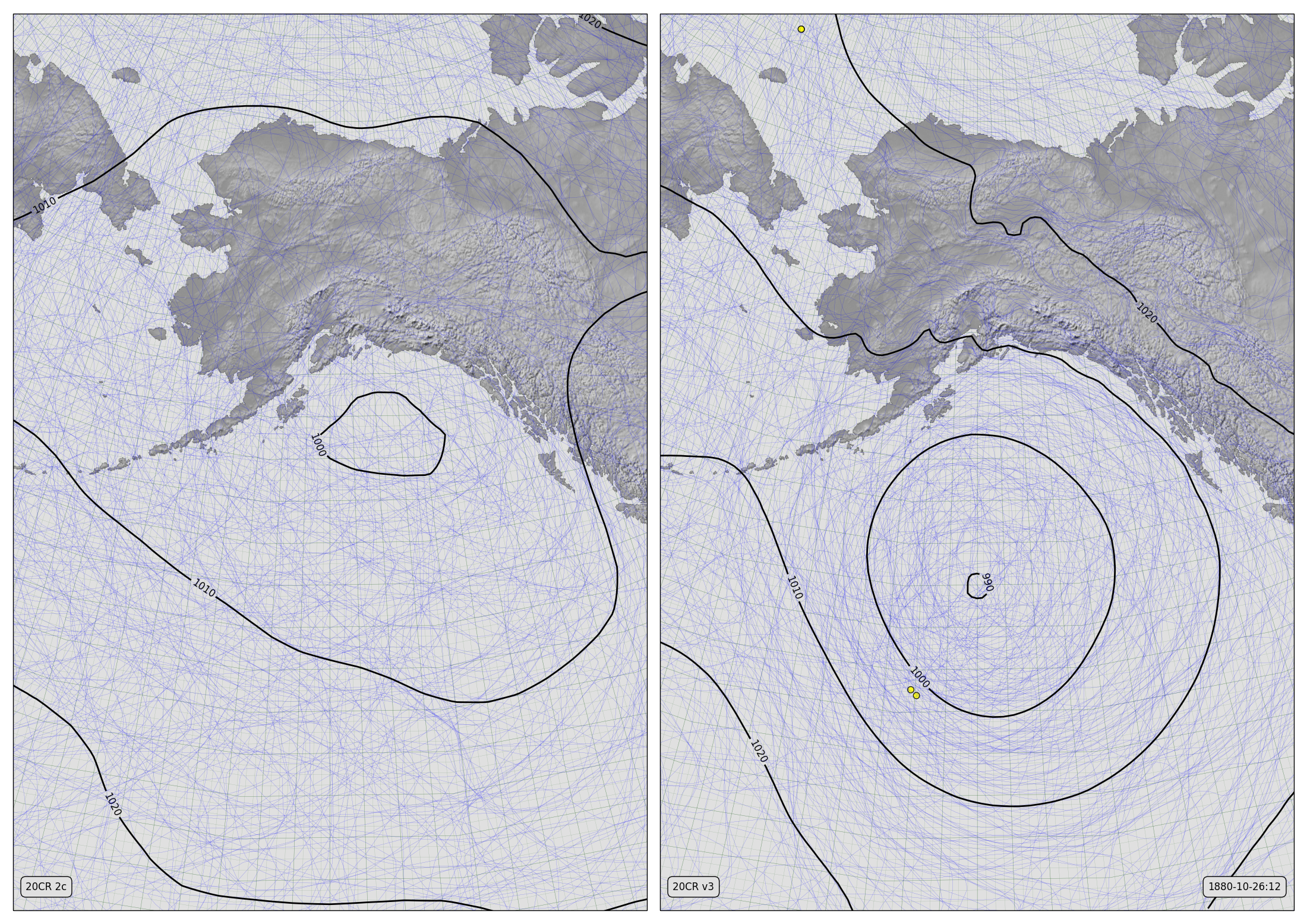# Sitka Hurricane (1880) - spinup version¶

Video versionMSLP Contours for v2c (left) and v3 (right)

The thin blue lines are mslp contours from each of 56 ensemble members (all members for v2c, the first 56 members for v3). The thicker black lines are contours of the ensemble mean. The yellow dots mark pressure observations assimilated while making the field shown.

20CRv3 reconstructed this event twice, one in the stream started in November 1879 (so October 1880 is still in the spinup period) - that’s this version. The version in the stream starting in November 1874 is not only fully spun-up, but also includes more observations: the observations from the USS Jamestown (at Sitka) were added to those from the Jeannette (Arctic Ocean) and Yukon (North Pacific) in this version.

The “Sitka Hurricane” was an unusually strong storm that made landfall near Sitka, in Alaska, on October 26, 1880.

Make the figure:

```#!/usr/bin/env python

# UK region weather plot
# Compare pressures from 20CRV3 and 20CRV2c

import math
import datetime
import numpy
import pandas

import iris
import iris.analysis

import matplotlib
from matplotlib.backends.backend_agg import \
FigureCanvasAgg as FigureCanvas
from matplotlib.figure import Figure

import cartopy
import cartopy.crs as ccrs

import Meteorographica as mg
import IRData.twcr as twcr

# Date to show
year=1880
month=10
day=26
hour=12
dte=datetime.datetime(year,month,day,hour)

# Landscape page
fig=Figure(figsize=(22,22/math.sqrt(2)),  # Width, Height (inches)
dpi=100,
facecolor=(0.88,0.88,0.88,1),
edgecolor=None,
linewidth=0.0,
frameon=False,
subplotpars=None,
tight_layout=None)
canvas=FigureCanvas(fig)

# North Pacific-centred projection
projection=ccrs.RotatedPole(pole_longitude=30, pole_latitude=35)
scale=15
extent=[scale*-1,scale,scale*-1*math.sqrt(2),scale*math.sqrt(2)]

# Two side-by-side plots
ax_2c.set_axis_off()
ax_2c.set_extent(extent, crs=projection)
ax_3.set_axis_off()
ax_3.set_extent(extent, crs=projection)

# Background, grid and land for both
ax_2c.background_patch.set_facecolor((0.88,0.88,0.88,1))
ax_3.background_patch.set_facecolor((0.88,0.88,0.88,1))
land_img_2c=ax_2c.background_img(name='GreyT', resolution='low')
land_img_3=ax_3.background_img(name='GreyT', resolution='low')

# Add the observations from 2c

# Contour spaghetti plot of ensemble members
mg.pressure.plot(ax_2c,prmsl,scale=0.01,type='spaghetti',
resolution=0.25,
levels=numpy.arange(870,1050,10),
colors='blue',
label=False,
linewidths=0.1)

# Add the ensemble mean - with labels
prmsl_m=prmsl.collapsed('member', iris.analysis.MEAN)
mg.pressure.plot(ax_2c,prmsl_m,scale=0.01,
resolution=0.25,
levels=numpy.arange(870,1050,10),
colors='black',
label=True,
linewidths=2)

# 20CR2c label
mg.utils.plot_label(ax_2c,'20CR 2c',
facecolor=fig.get_facecolor(),
x_fraction=0.02,
horizontalalignment='left')

# V3 panel

# Add the observations from v3

# Contour spaghetti plot of ensemble members
# Only use 56 members to match v2c
prmsl_r=prmsl.extract(iris.Constraint(member=list(range(0,56))))
mg.pressure.plot(ax_3,prmsl_r,scale=0.01,type='spaghetti',
resolution=0.25,
levels=numpy.arange(870,1050,10),
colors='blue',
label=False,
linewidths=0.1)

# Add the ensemble mean - with labels
prmsl_m=prmsl.collapsed('member', iris.analysis.MEAN)
mg.pressure.plot(ax_3,prmsl_m,scale=0.01,
resolution=0.25,
levels=numpy.arange(870,1050,10),
colors='black',
label=True,
linewidths=2)

mg.utils.plot_label(ax_3,'20CR v3',
facecolor=fig.get_facecolor(),
x_fraction=0.02,
horizontalalignment='left')

mg.utils.plot_label(ax_3,
'%04d-%02d-%02d:%02d' % (year,month,day,hour),
facecolor=fig.get_facecolor(),
x_fraction=0.98,
horizontalalignment='right')

# Output as png
fig.savefig('V3vV2c_Sitka_Hurricane_%04d%02d%02d%02d.png' %
(year,month,day,hour))
```